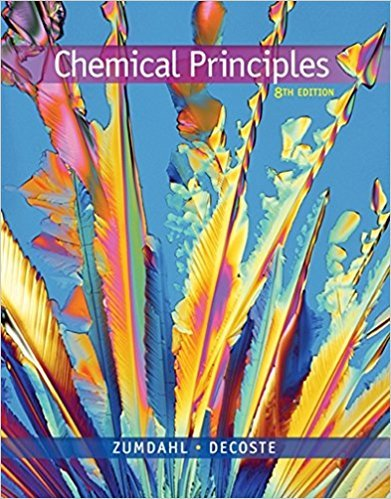×
Get Full Access to Chemical Principles - 8 Edition - Chapter 10 - Problem 10.37
Get Full Access to Chemical Principles - 8 Edition - Chapter 10 - Problem 10.37

×

# Calculate the change in entropy that occurs when 18.02 gISBN: 9781305581982 176

## Solution for problem 10.37 Chapter 10

Chemical Principles | 8th Edition

• Textbook Solutions
• 2901 Step-by-step solutions solved by professors and subject experts
• Get 24/7 help from StudySoup virtual teaching assistantsChemical Principles | 8th Edition

4 5 1 296 Reviews
25
2
Problem 10.37

Calculate the change in entropy that occurs when 18.02 g of ice at 210.08C is placed in 54.05 g of water at 100.08C in a perfectly insulated vessel. Assume that the molar heat capacities for H2O(s) and H2O(l) are 37.5 J K21 mol21 and 75.3 J K21 mol21, respectively, and the molar enthalpy of fusion for ice is 6.01 kJ/mol.

Step-by-Step Solution:

Problem 10.37

Calculate the change in entropy that occurs when 18.02 g of ice at -10.0°C is placed in 54.05 g of water at 100.0°C in a perfectly insulated vessel. Assume that the molar heat capacities for H2O(s) and H2O(l) are 37.5 J K-1 mol21 and 75.3 J K-1 mol-1, respectively, and the molar enthalpy of fusion for ice is 6.01 kJ/mol.

Step by step solution

Step 1 of 4

The heat that is used to heat up the ice, melt it, and heat up the resulting liquid will come from

the warm water.

Let’s, calculate the molar amount of each source of water.Step 2 of 4

Step 3 of 4

##### ISBN: 9781305581982

Chemical Principles was written by and is associated to the ISBN: 9781305581982. This textbook survival guide was created for the textbook: Chemical Principles, edition: 8. This full solution covers the following key subjects: . This expansive textbook survival guide covers 20 chapters, and 2754 solutions. Since the solution to 10.37 from 10 chapter was answered, more than 1413 students have viewed the full step-by-step answer. The full step-by-step solution to problem: 10.37 from chapter: 10 was answered by , our top Chemistry solution expert on 12/23/17, 04:53PM. The answer to “Calculate the change in entropy that occurs when 18.02 g of ice at 210.08C is placed in 54.05 g of water at 100.08C in a perfectly insulated vessel. Assume that the molar heat capacities for H2O(s) and H2O(l) are 37.5 J K21 mol21 and 75.3 J K21 mol21, respectively, and the molar enthalpy of fusion for ice is 6.01 kJ/mol.” is broken down into a number of easy to follow steps, and 60 words.

Unlock Textbook Solution